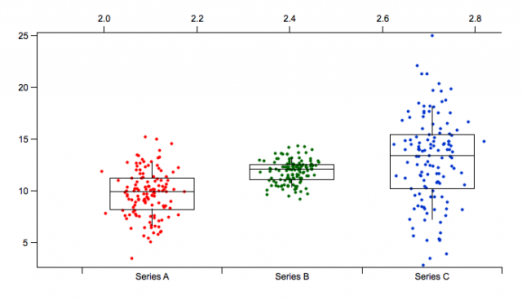Data Points added to Box PlotsI think there is an opportunity to improve the box plot graphs by adding the data points from the series.

The layout is similar to that produced in JMP and I have found that the addition of the data points to the box plots very useful.

In the attached example I overlayed the box and whisker on top of the datapoints. For Series A, the label is set at X=0, Series B at x = 1, and Series C at x =2. I created a wave for the x values for each series with x = 0 + gnoise(.3), x = 1 + gnoise(.3).... and then plotted the series values versus the x value waves. The reason for the jitter is to show points that may overlap because they have the same value.

This adds another dimension to looking at the data. I think it would be an easy addition. Everything for the folks at Wavemetrics is easy.Update: The ability to do this sort of thing is present in 6.2. From the what's new file:

Added /NCAT flag to AppendToGraph. Causes trace to be plotted normally on what otherwise is a category plot. x values are just category numbers but can be fractional. Category numbers start from zero. This can be used to overlay the original data points for a box plot. Here are examples:

Make/T/O catx={"cat0", "cat1", "cat2"}
Make/O caty= { 1, 3, 2}
Display caty vs catx
SetAxis/A/E=1 left

// Example 1: plot a function vertically overlaying a category
Make/O cat0over= 0.5*(sin(p/8)+1)
SetScale x, 0,3,cat0over
AppendToGraph/NCAT/VERT cat0over

// Example 2: simulate original data for a box plot
Make/N=10/O cat1over= gnoise(1)+1.5
SetScale/P x, 1.5, 1e-5, cat1over   // delta x can not be zero
AppendToGraph/NCAT cat1over
ModifyGraph mode(cat1over)=3,marker(cat1over)=19,rgb(cat1over)=(0,0,65535)

Dear Dr. Hededus,

I am a very naive beginner of the use of Igor, and I found your fascinating post about Data points added to Box plots. I would really appreciate if you can teach me how to create this graph.